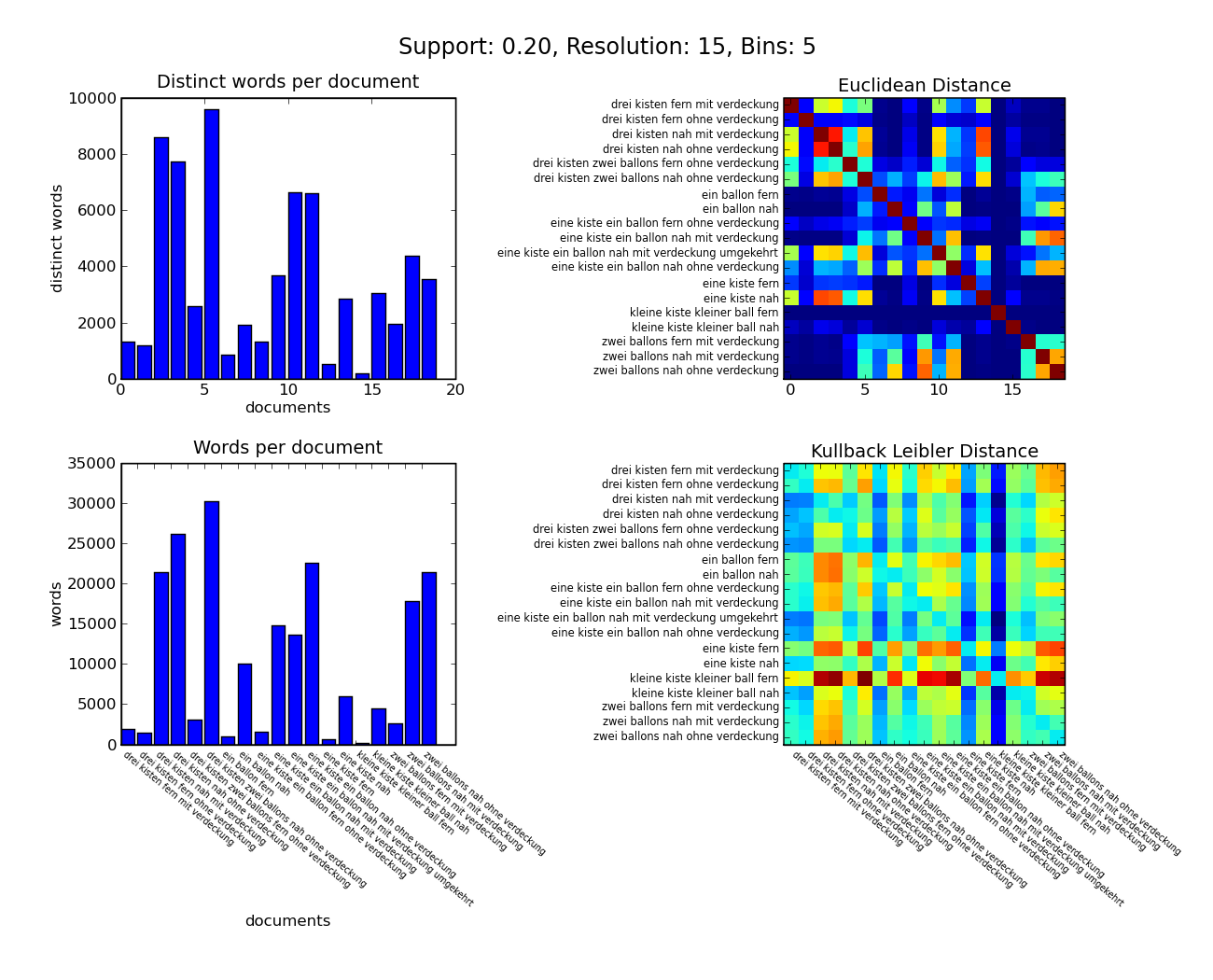# ColorBar with axes

Hello,

I would like to show a colorbar for my plot (see attachment) but I can't
figure out how it works.
In the examples on the website I only found the call to pylab.colorbar(),
which doesn't work with my subplots. My code is as follows (with the bar
graph-part snipped, for brevity). I also tried the ColorBar class and
assigning "cmap=cm.jet" but it didn't work. Could anybody give me a hint
please? I searched the tutorial, cookbook, api reference and google, but I
can't really find anything.

Thanks,
Felix

fig = p.figure()
fig.text(0.5, 0.94, main_title, fontsize="x-large", ha="center")

(...bar graph part snipped...)

ax.set_title('Euclidean Distance')
matrix = n.zeros([numof_dicts, numof_dicts])
for i1, d1 in enumerate(dictionaries):
for i2, d2 in enumerate(dictionaries):
for k in d1.keys():
if d2.has_key(k):
prob1 = (d1[k] / vectorlengths[i1])
prob2 = (d2[k] / vectorlengths[i2])
matrix[i1, i2] += prob1 * prob2
ax.imshow(matrix, interpolation="nearest", cmap=p.cm.jet)
if documentnames != None:
ax.set_yticklabels(documentnames, va="center", fontsize='x-small')
ax.set_yticks(range(numof_dicts))
#p.colorbar()

ax.set_title('Kullback Leibler Distance')
ax.imshow(matrix, interpolation="nearest")

if documentnames != None:
ax.set_yticklabels(documentnames, va="center", fontsize='x-small')
ax.set_yticks(range(numof_dicts))
ax.set_xticklabels(documentnames, rotation=320, va="top", ha="left",
fontsize='xx-small')
ax.set_xticks(range(numof_dicts))

hspace=0.3,wspace=0.9)
fig.set_size_inches(13, 10.5)
fig.savefig(sys.argv + "_statistics.png")
#p.show()felix@...2111... wrote:

Hello,

I would like to show a colorbar for my plot (see attachment) but I can't
figure out how it works.
In the examples on the website I only found the call to pylab.colorbar(),
which doesn't work with my subplots. My code is as follows (with the bar
graph-part snipped, for brevity). I also tried the ColorBar class and
assigning "cmap=cm.jet" but it didn't work. Could anybody give me a hint
please? I searched the tutorial, cookbook, api reference and google, but I
can't really find anything.

Thanks,
Felix

fig = p.figure()
fig.text(0.5, 0.94, main_title, fontsize="x-large", ha="center")

(...bar graph part snipped...)

ax.set_title('Euclidean Distance')
matrix = n.zeros([numof_dicts, numof_dicts])
for i1, d1 in enumerate(dictionaries):
for i2, d2 in enumerate(dictionaries):
for k in d1.keys():
if d2.has_key(k):
prob1 = (d1[k] / vectorlengths[i1])
prob2 = (d2[k] / vectorlengths[i2])
matrix[i1, i2] += prob1 * prob2
ax.imshow(matrix, interpolation="nearest", cmap=p.cm.jet)

im = ax.imshow(matrix, interpolation="nearest", cmap=p.cm.jet)
p.colorbar(im, ax=ax)

Try the above, possibly adding a "shrink" kwarg; if you don't like the axes splitting that colorbar does by default, then you can make an axes to taste, call it "cax", and instead of specifying the image axes as above, use p.colorbar(im, cax=cax).

Eric

···

if documentnames != None:
ax.set_yticklabels(documentnames, va="center", fontsize='x-small')
ax.set_yticks(range(numof_dicts))
#p.colorbar()

ax.set_title('Kullback Leibler Distance')
ax.imshow(matrix, interpolation="nearest")

if documentnames != None:
ax.set_yticklabels(documentnames, va="center", fontsize='x-small')
ax.set_yticks(range(numof_dicts))
ax.set_xticklabels(documentnames, rotation=320, va="top", ha="left",
fontsize='xx-small')
ax.set_xticks(range(numof_dicts))